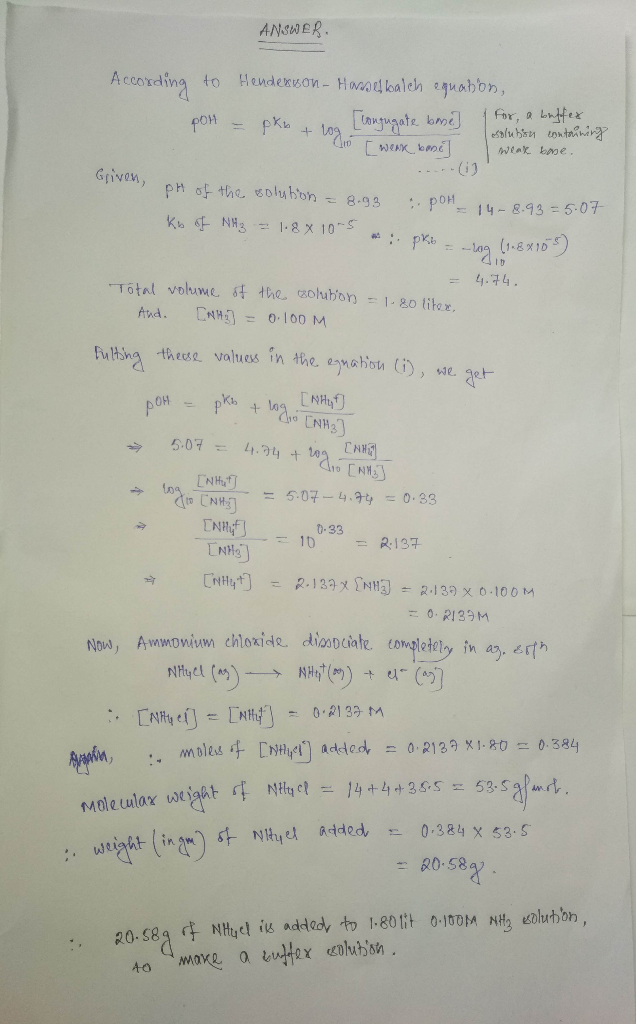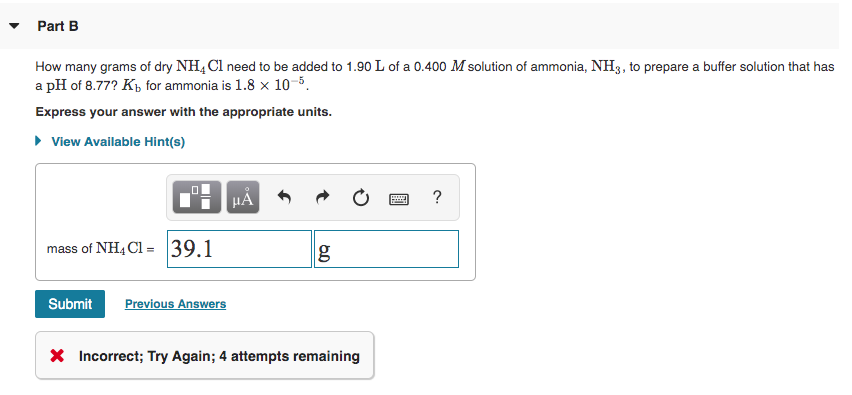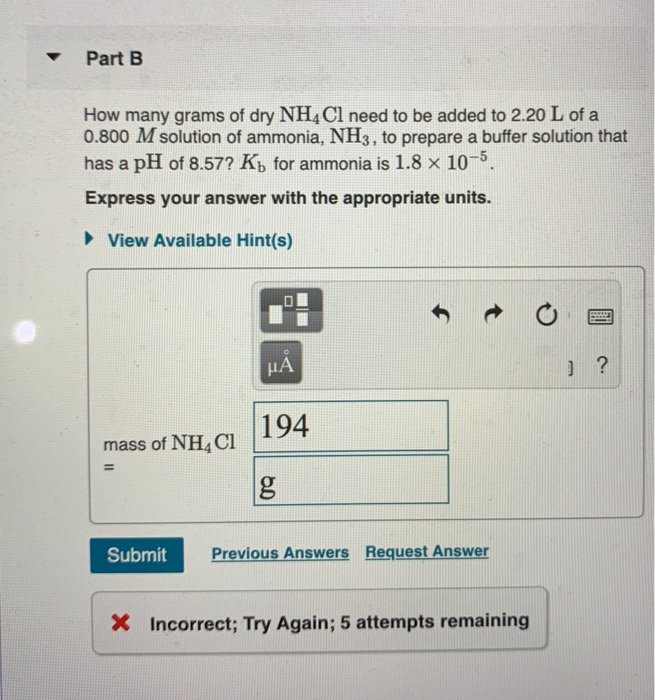Question

# 4B. How many grams of dry NH4Cl need to be added to 1.80 L of a...

4B. How many grams of dry NH4Cl need to be added to 1.80 L of a 0.100 M solution of ammonia, NH3, to prepare a buffer solution that has a pH of 8.93? Kb for ammonia is 1.8×10^−5. Express your answer with the appropriate units.#### Earn Coins

Coins can be redeemed for fabulous gifts.

Similar Homework Help Questions
• ### How many grams of dry NH4Cl need to be added to 2.00 L of a 0.700...

How many grams of dry NH4Cl need to be added to 2.00 L of a 0.700 M solution of ammonia, NH3, to prepare a buffer solution that has a pH of 9.00? Kb for ammonia is 1.8×10−5. Express your answer with the appropriate units.

• ### How many grams of dry NH4Cl need to be added to 1.90 L of a 0.800...

How many grams of dry NH4Cl need to be added to 1.90 L of a 0.800 M solution of ammonia, NH3, to prepare a buffer solution that has a pH of 8.74? Kb for ammonia is 1.8×10^−5. Express your answer with the appropriate units.

• ### How many grams of dry NH4Cl need to be added to 2.10 L of a 0.500 M solution of ammonia, NH3, to prepare a buffer solu...

How many grams of dry NH4Cl need to be added to 2.10 L of a 0.500 M solution of ammonia, NH3, to prepare a buffer solution that has a pH of 8.76? Kb for ammonia is 1.8×10−5. Express your answer with the appropriate units.

• ### How many grams of dry NH4Cl need to be added to 1.50 L of a 0.700...

How many grams of dry NH4Cl need to be added to 1.50 L of a 0.700 M solution of ammonia, NH3, to prepare a buffer solution that has a pH of 8.56? Kb for ammonia is 1.8×10−5.

• ### How many grams of dry NH4Cl need to be added to 1.90 L of a 0.800...

How many grams of dry NH4Cl need to be added to 1.90 L of a 0.800 M solution of ammonia, NH3, to prepare a buffer solution that has a pH of 8.74? Kb for ammonia is 1.8×10^-5.

• ### How many grams of dry NH4Cl need to be added to 1.70 L of a 0.100 M solution of ammonia, NH3, to prepare a buffer solu...

How many grams of dry NH4Cl need to be added to 1.70 L of a 0.100 M solution of ammonia, NH3, to prepare a buffer solution that has a pH of 8.62? Kb for ammonia is 1.8×10−5.

• ### Part B How many grams of dry NH4Cl need to be added to 1.90 L of...Part B How many grams of dry NH4Cl need to be added to 1.90 L of a 0.400 M solution of ammonia, NH3, to prepare a buffer solution that has a pH of 8.77? Kb for ammonia is 1.8 x 10-5 Express your answer with the appropriate units. View Available Hint(s) CHÅN O a? mass of NH4C1 = 39.1 g Submit Previous Answers X Incorrect; Try Again; 4 attempts remaining

• ### How many grams of dry NH4Cl need to be added to 2.30 L of a 0.100 M solution of ammonia,NH3, to prepare a buffer soluti...

How many grams of dry NH4Cl need to be added to 2.30 L of a 0.100 M solution of ammonia,NH3, to prepare a buffer solution that has a pH of 9.00? Kb for ammonia is 1.8x 10^-5.

• ### - Part B How many grams of dry NH4Cl need to be added to 2.20 L...- Part B How many grams of dry NH4Cl need to be added to 2.20 L of a 0.800 M solution of ammonia, NH3, to prepare a buffer solution that has a pH of 8.57? Ký for ammonia is 1.8 x 10-5. Express your answer with the appropriate units. View Available Hint(s) UA 194 mass of NHACI Submit Previous Answers Request Answer X Incorrect; Try Again; 5 attempts remaining

• ### How many grams of dry NH4Cl need to be added to 2.50 L of a 0.500 M solution of ammonia, NH3, to prepare a buffer soluti...

How many grams of dry NH4Cl need to be added to 2.50 L of a 0.500 M solution of ammonia, NH3, to prepare a buffer solution that has a pH of 8.74? Kb for ammonia is 1.8*10^-5.